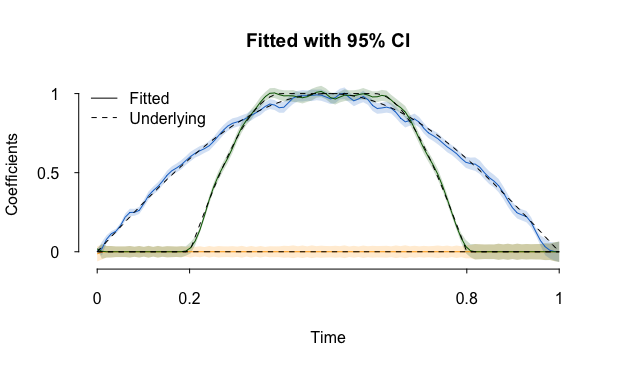This package implements paper “Functional Group Bridge for Simultaneous Regression and Support Estimation”

[PDF] [Github] [CRAN]## Installation

You can install the released version of spfdafrom CRAN with:

install.packages("spfda")

The experimental version can be installed via

# install.packages("remotes")
remotes::install_github("dipterix/spfda")

## Example

library(spfda)
dat <- spfda_simulate()
x <- dat$X y <- dat$Y

## basic example code

fit <- spfda(y, x, lambda = 5, CI = TRUE)

## Generics

BIC(fit)
plot(fit, col = c("orange", "dodgerblue3", "darkgreen"),
main = "Fitted with 95% CI", aty = c(0, 0.5, 1), atx = c(0,0.2,0.8,1))
matpoints(fit$time, t(dat$env\$beta), type = 'l', col = 'black', lty = 2)
legend('topleft', c("Fitted", "Underlying"), lty = c(1,2), bty = 'n')
print(fit)
coefficients(fit)

## Citation

Use citation('spfda') to generate citation information, or check this link.

Wang, Z, Magnotti, JF, Beauchamp, MS. Li, M, Functional Group Bridge for
Simultaneous Regression and Support Estimation.